# Preface

This section is about Fourier series and its basic properties. Further discussions and its applications are presented in the following sections.

Introduction to Linear Algebra with Mathematica

Although there are many methods for signal representations, Fourier analysis involving the resolution of signals into sinusoidal components dominates. Periodic signals occur in a wide range of physical phenomena, a few examples of which are acoustic and electromagnetic waves, periodic vibrations of musical instruments, vertical displacement of a mechanical pendulum, and periodic signals in electrical engineering.

This section presents a particular application of eigenfunction expansion corresponding to the Sturm--Liouville problem with periodic conditions,

$L_2 \left[ \texttt{D} \right] y = \lambda\,y(x) \qquad \Longleftrightarrow \qquad y'' +\lambda\,y(x) = 0 , \qquad y(-\ell ) = y(\ell ) ,$
where ℓ is a positive number and $$L_2 \left[ \texttt{D} \right] = - \texttt{D}^2$$ is the second order differential operator with $$\texttt{D} = {\text d}/{\text d}x .$$ The problem assumes that its solution y(x) is a real-valued function defined on a symmetric interval (for simplicity) of length 2ℓ. Because of periodically condition, the function y(x) can be considered only on this finite interval. Instead of the second order differential operator L2, it is convenient to consider its equivalent form written in terms of complex-valued functions:
$L_1 \left[ \texttt{D} \right] u = \mu\, u(x) \qquad \Longleftrightarrow \qquad {\bf j}\,u' + \mu\, u(x) =0, \qquad u(-\ell ) = u(\ell ) ,$
where $$L_1 \left[ \texttt{D} \right] = - {\bf j}\,\texttt{D}$$ is the first order differential operator and j is the unit vector along positive vertical direction on the complex plane ℂ, so j² = −1. Coefficients ck of an expansion, $$f(x) = \sum_k c_k \phi_k (x) ,$$ for a function f(x) over the set of eienfunctions {ϕk(x)} are called the Fourier coefficients. Restoring the function from its Fourier coefficients is an ill-posed problem.
The Sturm--Liouville problems formulated above can be written in operator form
$L \left[ \texttt{D} \right] y = \lambda\,y , \qquad y(-\ell ) = y(\ell ) ,$
where
$L_2 \left[ \texttt{D} \right] = - \texttt{D}^2 \qquad \mbox{or} \qquad L_1 \left[ \texttt{D} \right] = -{\bf j}\,\texttt{D} , \qquad\mbox{with} \quad \texttt{D} = {\text d}/{\text d}x .$
Actually, these two differential operators are related:
$L_2 \left[ \texttt{D} \right] = L_1 \left[ \texttt{D} \right]^2 \qquad \mbox{or} \qquad - \texttt{D}^2 = \left( - {\bf j}\,\texttt{D} \right)^2 = {\bf j}^2 \texttt{D}^2 .$
As it was shown in the previous section, the unbounded linear operator L2 with periodic conditions is a non-negative operator, meaning that all its eigenvalues are not negative.

Any eigenvalue problem can be written in abstract way as $$L\, y = \lambda\,y ,$$ which is actually a spectral representation of the linear operator L. The idea of such representation is to reduce action of the operator L on a function to multiplication operation. In contrast to finite dimensional case (matrices), an unbounded operator has infinite many (maybe continuous) eigenvalues.

Assuming λ≥0, we solve the Sturm--Liouville problem for L2.

$y'' + \lambda\,y = 0, \qquad y(-\ell ) = y(\ell ) .$
The general solution of the differential equation depends on two arbitrary real constants c1 and c2:
$y(x) = c_1 \cos \left( \sqrt{\lambda}\,x \right) + c_2 \sin \left( \sqrt{\lambda}\,x \right) .$
This function can be periodic with period T if and only if
$\sqrt{\lambda} = \frac{2\pi n}{T} \qquad \Longrightarrow \qquad \lambda_n = \left( \frac{2\pi n}{T} \right)^2 , \quad n=0,1,2,\ldots .$
It is a custom to set the period to be an even number: T = 2ℓ. Then due to periodicity of the problem, it is also convenient to consider a symmetric interval [−ℓ, ℓ] because any interval of length T = 2ℓ can be used.

The first order differential operator L1 acts on complex-valued functions (as well as on real-valued functions). Its general solution depends on one arbitrary complex number A = 𝑎 + jb (where 𝑎 and b are real numbers):

$u(x) = A\, e^{{\bf j}\mu x} = \left( a + {\bf j}b \right) e^{{\bf j}\mu x} = \left( a + {\bf j}b \right) \left( \cos \mu x + {\bf j}\,\sin \mu x \right) .$
The function u(x) is a periodic function (independently on A) when
$\mu = \mu_n = \frac{n2\pi}{T} , \qquad n= 0,1,2,\ldots .$
Note that since L2 = L1², their eigenvalues are related
$\lambda_n = \mu_n^2 = \left( \frac{2\pi n}{T} \right)^2 , \quad n=0,1,2,\ldots .$
Finally, we get a sequence of eigenfunctions corresponding to eigenvalues μn:
$u_n (x) = A_n e^{2{\bf j}n\pi x/T} , \qquad \mbox{where} \quad T = 2\ell .$

﹡ ⁎ ✱ ✲ ✳ ✺ ✻ ✼ ✽ ❋

# Fourier Series

We start with the classical Fourier expansion of a periodic function and then present some important expansions that are widely used in applications. We will assume that all periodic functions have period 2ℓ.

There are two equivalent forms of Fourier series based on two Sturm--Liouville problems for the first order operator $$L_1 \left[ \texttt{D} \right] = - {\bf j}\,\texttt{D}$$ and the second order operator $$L_2 \left[ \texttt{D} \right] = - \texttt{D}^2 .$$ The former one provides an eigenfunction expansion in a complex form

\begin{equation} \label{EqFourier.1} f(x) \,\sim\, \sum_{k=-\infty}^{\infty} \alpha_k e^{k{\bf j} \pi x/\ell} , \end{equation}
where (T = 2ℓ)
\begin{equation} \label{EqFourier.2} \alpha_k = \alpha_k (f) = \frac{1}{2\ell} \int_{-\ell}^{\ell} f(x)\, e^{-k{\bf j} \pi x/\ell} \,{\text d} x = \frac{1}{T} \int_{0}^{T} f(x)\, e^{-2k{\bf j} \pi x/T} \,{\text d} x , \qquad k=0, \pm 1, \pm 2, \ldots . \end{equation}
Another eigenfunction expansion regarding the second order differential operator L2 gives a trigonometric form
\begin{equation} \label{EqFourier.3} f(x) \,\sim\, \frac{a_0}{2} + \sum_{k=1}^{\infty} \left[ a_k \cos \frac{k \pi x}{\ell} + b_k \sin \frac{k \pi x}{\ell} \right] , \end{equation}
where coefficients are defined according to the Euler--Fourier formulas (they were discovered in 1777 by Leonhard Euler many years before Fourier used them in his research):
\begin{align} a_0 &= a_0 (f) = \frac{1}{\ell} \int_{-\ell}^{\ell} f(x)\,{\text d} x , \notag \\ a_k &= a_k (f) = \frac{1}{\ell} \int_{-\ell}^{\ell} f(x)\, \cos \frac{k \pi x}{\ell} \,{\text d} x , \qquad k= 1, 2, 3, \ldots ; \label{EqFourier.4} \\ b_k &= b_k (f) = \frac{1}{\ell} \int_{-\ell}^{\ell} f(x)\, \sin \frac{k \pi x}{\ell} \,{\text d} x , \qquad k= 1, 2, 3, \ldots . \notag \end{align}
Coefficient evaluations in the Euler--Fourier formulas \eqref{EqFourier.2} and \eqref{EqFourier.4} can be obtained upon integration over any interval of length T = 2ℓ because of periodically condition. Sometimes it is convenient to consider a function on interval [0,T] (or any interval of length T). Let f(x) be a periodic function over the range 0 ≤ x < T. Then its Fourier series becomes
\begin{equation} \label{EqFourier.5} f(x) \,\sim\, \frac{a_0}{2} + \sum_{k\ge 1} \left[ a_k \cos \frac{2k \pi x}{T} + b_k \sin \frac{2k \pi x}{T} \right] , \end{equation}
where coefficients are defined as
\begin{equation} \label{EqFourier.5T} \begin{split} a_k &= \frac{2}{T} \int_{0}^{T} f(x)\, \cos \frac{2k \pi x}{T} \,{\text d} x , \qquad k= 0, 1, 2, 3, \ldots ; \\ b_k &= \frac{2}{T} \int_{0}^{T} f(x)\, \sin \frac{2k \pi x}{T} \,{\text d} x , \qquad k= 1, 2, 3, \ldots . \end{split} \end{equation}

All expansion formulas involve infinite series; their convergence is based on properties of partial sums, either \eqref{EqFourier.3}

$S_N (x) = \frac{a_0}{2} + \sum_{k=1}^{N} \left[ a_k \cos \frac{k \pi x}{\ell} + b_k \sin \frac{k \pi x}{\ell} \right] ,$
for real-valued functions, or \eqref{EqFourier.1}
$\mbox{P.V. } \ S_N (x) = \sum_{k=-N}^{N} \alpha_k e^{k{\bf j} \pi x/\ell}$
for complex-valued functions, where P.V. stands for the Cauchy principal value. A Fourier series converges when its partial sums SN(x) approach a limit in some sense; it exists not for arbitrary functions. Since a Fourier series is a series involving trigonometric functions, the corresponding partial sums depend on a parameter x∈ℝ. Upon considering partial sums for every value of x, we arrive at the pointwise convergence of the series of functions (in our case, trigonometric functions). A pointwise convergence of Fourier series is a very delicate matter, which we touch in convergence section.

A natural question that comes to your mind is whether the series \eqref{EqFourier.1} or \eqref{EqFourier.5} converges and if they converge, do they converge to generating function f(x)? Mathematicians tell us that none of these questions have simple answers. Nonetheless, Fourier series usually work quite well (especially in situations where they arise from physical problems).

Although we don't know necessary and sufficient conditions for a function to be expanded into a Fourier series, we know some sufficient conditions that guarantee a Fourier series expansion. The Fourier series does not exist unless, for example, 𝑎0 exists (i.e., $$\left\vert \int_{-\ell}^{\ell} f(x) \,{\text d}x \right\vert < \infty$$ ). Even when the integral for 𝑎0 exists, the Fourier series may converge to another function. Therefore, we prefer to use the tilde notation (∼) to indicate that function f(x) is used to evaluate Fourier coefficients and build a corresponding series. The symbol ∼ is read as “has the Fourier series (on a given interval).” If we know that the Fourier series converges to f(x), then tilde is replaced by the equal sign (=).

Example 1: Consider a function f(x) = x² on the interval [0,2]. Its Fourier coefficients can be determined with Mathematica:

Integrate[x^2, {x, 0, 2}]
8/3
Simplify[Integrate[x^2 * Cos[n*Pi*x], {x, 0, 2}], Assumptions -> Element[n, Integers]]
4/(n^2 $Pi]^2) Simplify[Integrate[x^2 * Sin[n*Pi*x], {x, 0, 2}], Assumptions -> Element[n, Integers]] 4/(n \[Pi]) Therefore, we get the following Fourier series for function x²: \[ x^2 = \frac{4}{3} + \sum_{n\ge 1} \left[ \frac{4}{n^2 \pi^2}\,\cos \left( n\pi x \right) - \frac{4}{n\,\pi}\,\sin \left( n\pi x \right) \right] .$
Next we plot some partial sums along with the given function x²
S10[x_] = 4/3 + Sum[4/n^2/Pi^2 *Cos[n*Pi*x] - 4/n/Pi*Sin[n*Pi*x], {n, 1, 10}];
S20[x_] = 4/3 + Sum[4/n^2/Pi^2 *Cos[n*Pi*x] - 4/n/Pi*Sin[n*Pi*x], {n, 1, 20}];
S100[x_] = 4/3 + Sum[ 4/n^2/Pi^2 *Cos[n*Pi*x] - 4/n/Pi*Sin[n*Pi*x], {n, 1, 100}];
Plot[{x^2, S10[x]}, {x, -0.5, 2.5}, PlotStyle -> {Thick, {Black, Red}}]
Plot[{x^2, S20[x]}, {x, -0.5, 2.5}, PlotStyle -> {Thick, {Black, Orange}}]
Plot[{x^2, S100[x]}, {x, -0.5, 2.5}, PlotStyle -> {Thick, {Black, Blue}}]
Fourier approximation with 10 terms    Fourier approximation with 20 terms    Fourier approximation with 100 terms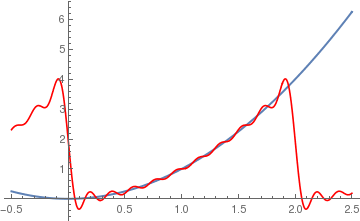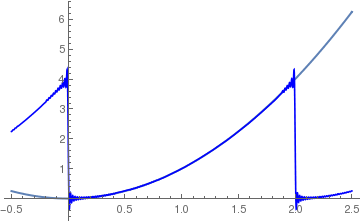Theorem 1: The following formulas could be helpful for Fourier evaluations ($$\texttt{D} = \texttt{D}_t = {\text d}/{\text d}t$$ is the derivative operator):
\begin{align*} \int t^n \cos (at) \,{\text d}t &= - \frac{1}{a} \sum_{k=0}^n \frac{1}{a^k} \, {\texttt D}_t^k \left( t^k \right) \left. {\texttt D}_z^{k+1} \left( \cos z \right) \right\vert_{z=at} \\ &= - \frac{1}{a} \left[ t^n {\texttt D}_z \cos z + \frac{n\,t^{n-1}}{a} \, {\texttt D}_z^2 \cos z + \cdots + \frac{n!}{a^n} \, {\texttt D}_z^{n+1} \cos z \right]_{z=at} , \\ \int t^n \sin (at) \,{\text d}t &= - \frac{1}{a} \sum_{k=0}^n \frac{1}{a^k} \, {\texttt D}_t^k \left( t^k \right) \left. {\texttt D}_z^{k+1} \left( \sin z \right) \right\vert_{z=at} \\ &= - \frac{1}{a} \left[ t^n {\texttt D}_z \sin z + \frac{n\,t^{n-1}}{a} \, {\texttt D}_z^2 \sin z + \cdots + \frac{n!}{a^n} \, {\texttt D}_z^{n+1} \sin z \right]_{z=at} .     \end{align*}

The complex Fourier series \eqref{EqFourier.1} is more elegant and shorter to write down than the one expressed in term of sines and cosines \eqref{EqFourier.3}, but it has the disadvantage that the coefficients might be complex numbers even if the given function is real-valued. The trigonometric form is based on Euler's formulas:

$\sin \theta = \frac{1}{2{\bf j}} \,e^{{\bf j}\theta} - \frac{1}{2{\bf j}} \,e^{-{\bf j}\theta} = \Im \,e^{{\bf j}\theta} = \mbox{Im} \,e^{{\bf j}\theta}, \qquad \cos \theta = \frac{1}{2} \,e^{{\bf j}\theta} - \frac{1}{2} \,e^{-{\bf j}\theta} = \Re \,e^{{\bf j}\theta} = \mbox{Re} \,e^{{\bf j}\theta}.$

Here, j is the unit vector in the positive vertical direction on the complex plane ℂ, so j² = −1. Mathematica has four default commands to calculate Fourier series:

FourierSeries        (* to calculate complex coefficient expansion *)
 FourierTrigSeries  (* to calculate standard Fourier expansion via sine and cosine *)
 FourierCosSeries (* to calculate cosine Fourier series *)
 FourierSinSeries   (* to calculate sine Fourier series *)

Sometimes, it is convenient to use polar form:

\begin{equation} \label{EqFourier.6} f(x) \,\sim\, \frac{a_0}{2} + \sum_{k=1}^{\infty} A_k \sin \left( \frac{k \pi x}{\ell} + \phi_k \right) = \frac{a_0}{2} + \sum_{k=1}^{\infty} A_k \cos \left( \frac{k \pi x}{\ell} - \varphi_k \right) , \end{equation}
where $$A_k = \sqrt{a_k^2 + b_k^2} \quad\mbox{and} \quad \varphi_k = \arctan \left( b_k / a_k \right) , \quad \phi_k = \arctan \left( a_k / b_k \right) .$$

In general, a square integrable function f ∈ 𝔏² on the interval [𝑎, b] of length b−𝑎 (b>𝑎) can be expanded into the Fourier series

\begin{equation} \label{EqFourier.7} f(x) \,\sim\, \frac{a_0}{2} + \sum_{k=1}^{\infty} a_k \cos \left( \frac{2x - a -b}{b-a} \right) + b_k \sin \left( \frac{2x - a -b}{b-a} \right) , \end{equation}
where
\begin{align*} a_k &= \frac{2}{b-a} \int_a^b f(x) \,\cos \left( \frac{2x - a -b}{b-a} \right) {\text d}x , \qquad k=0,1,2,\ldots , \\ b_k &= \frac{2}{b-a} \int_a^b f(x) \,\sin \left( \frac{2x - a -b}{b-a} \right) {\text d}x , \qquad k=1,2,\ldots . \end{align*}

For a real-valued periodic function f ∈ 𝔏²(−ℓ,ℓ), the following Parseval identity holds:

\begin{equation} \label{EqFourier.8} \frac{1}{\ell} \int_{-\ell}^{\ell} f^2 (x) \,{\text d}x = \frac{1}{2}\,a_0^2 + \sum_{k\ge 1} \left( a_k^2 + b_k^2 \right) . \end{equation}
For a complex Fourier series \eqref{EqFourier.1}, we have
\begin{equation} \label{EqFourier.9} \frac{1}{2\ell} \int_{-\ell}^{\ell} \left\vert f (x) \right\vert^2 {\text d}x = \sum_{k=-\infty}^{\infty} \left\vert \alpha_k \right\vert^2 . \end{equation}

Every Fourier series, either complex \eqref{EqFourier.1} or trigonometric {EqFourier.3} defines a sequence {αn}n∈ℤ of complex numbers or a sequence of pairs of real numbers {(𝑎k, bk}k∈ℕ, respectively. The Parseval identity tells us that that these sequences of numbers should belong to the complete space of sequences in its norm, denoted as ℓ²(ℤ) or ℓ²(ℕ), that consist of all sequences for which

$\| \left\{ \alpha_n \right\} \|_2 = \sum_{k=-\infty}^{\infty} \left\vert \alpha_k \right\vert^2 < \infty \qquad\mbox{or} \qquad \| \left\{ \left( a_k , b_k \right) \right\} \|_2 = \frac{1}{2}\,a_0^2 + \sum_{k\ge 1} \left( a_k^2 + b_k^2 \right) < \infty ,$ respectively. Here ℤ = { 0, ±1, ±2, … } is the set of all integers and ℕ = { 0, 1, 2, … } is the set of all nonnegative integers. However, not every sequence {αn}n∈ℤ from ℓ²(ℤ) defines a function that is square integrable in Riemann sense. It turns out that the Fourier series \eqref{EqFourier.1} defines a function that is Lebesgue square integrable.

Not every function is suitable for a Fourier series expansion, but those that satisfy some conditions. For example, the tangent function tan(x) cannot be expanded into the Fourier series on any interval containing the roots of the equation cos(x) = 0 simply because the Fourier coefficients do not exist. A German mathematician Paul David Gustav du Bois-Reymond (1831--1889) was able in 1873 to construct a continuous function whose Fourier series diverges at a point. Andrey Kolmogorov (1903--1987) from Moscow University (Russia), as a student at the age of 19, in his very first scientific work, constructed an example of an absolutely integrable function whose Fourier series diverges almost everywhere (later improved to diverge everywhere). We still do not know the exact conditions to fully identify the class of functions whose Fourier series pointwise converge to the same functions.

Before we get into the topic of convergence, we need to define some classes of functions that are sufficient for Fourier expansions and wide enough to include the majority of practical applications. First, we extend the set of continuous functions on the interval [−ℓ, ℓ], which is usually denoted as C[−ℓ, ℓ], that have an additional condition f(−ℓ) = f(ℓ). We need to consider piecewise continuous functions that consist of finite number of continuous pieces. We say that f(x) has a jump discontinuity at x = 𝑎 if the limit of the function from the left, $$\lim_{t\uparrow a} f(t) ,$$ denoted $$f(a-0) \ \mbox{ or }\ f(a^{-})$$ and the limit of the function from the right, $$\lim_{t\downarrow a} f(t) ,$$ denoted $$f(a+0) \ \mbox{ or }\ f(a^{+}) ,$$ both exist and $$f(a+0) \ne f(a-0) .$$ A function f(x) is called piecewise continuous on an interval [𝑎, b], if it is continuous on [𝑎, b] except for at most finitely many points $$x_1 , x_2 , \ldots , x_n$$ at each of them the function has both the left-hand and right-hand limits: $$f(x_k -0) \ \mbox{ and }\ f(x_k +0) , \quad k=1,2,\ldots , n .$$ Now we give the formal definition.

Let 𝑎 and b be real numbers such that 𝑎 < b. A function $$f\, : \, [a,b] \mapsto \,\mathbb{R}$$ is said to be piecewise continuous on $$[a,b]$$ if the following conditions are satisfied:
• there exists a finite set $$\{ x_1 , x_2 , \ldots , x_n \} \subset (a, b)$$ such that $$x_1 < x_2 < \cdots < x_n$$ and f is continuous and monotone on each subinterval
$\left( a , x_1 \right) , \quad \left( x_k , x_{k+1} \right) , \quad k=1,2,\ldots , n-1, \quad \left( x_n , b \right) ;$
• all the following one-sided limits exist
$\lim_{x\downarrow a} f \left( x \right) , \quad \lim_{x\uparrow x_k} f\left( x \right) , \quad \lim_{x\downarrow x_k} f\left( x \right) ,\quad k=1,2,\ldots , n-1, \quad \lim_{x \uparrow b} f\left( x \right) .$
A function $$f\, : \, \mathbb{R} \mapsto \,\mathbb{R}$$ is piecewise continuous on $$\mathbb{R} ,$$ if it is piecewise continuous on every finite subinterval of $$\mathbb{R} .$$

Example 2: The function

$f(x) = \begin{cases} 2x , & \ \mbox{ if } 0 < x < 1, \\ 1, & \ \mbox{ if } 1 < x < 2, \end{cases}$
is piecewise continuous on [0,2], but not continuous on [0,2].
f[x_]=Piecewise[{{2*x, 0<x<1},{1,1<x<2}}] Integrate[f[x], {x, 0, 2}]
2
Simplify[Integrate[f[x]*Cos[n*Pi*x], {x, 0, 2}], Assumptions -> Element[n, Integers]]
(2 (-1 + (-1)^n))/(n^2 $Pi]^2) Simplify[Integrate[f[x]*Sin[n*Pi*x], {x, 0, 2}], Assumptions -> Element[n, Integers]] -((1 + (-1)^n)/(n \[Pi])) Therefore, we get the following Fourier series for function x²: \[ f(x) = 1 + \sum_{n\ge 1} \left[ \frac{(-1)^n -1}{n^2\pi^2 /2} \,\cos \left( n\pi x \right) - \frac{(-1)^n +1}{n\pi} \,\sin \left( n\pi x \right) \right] .$
Next, we plot partial sums along with the given function.
Fourier approximation with 10 terms    Fourier approximation with 20 terms    Fourier approximation with 100 terms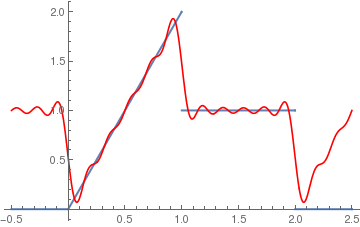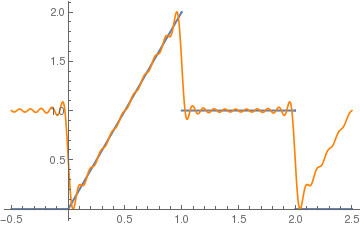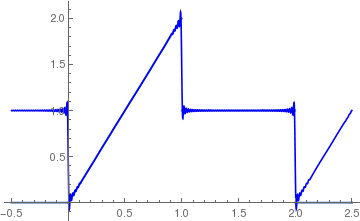Since the class of continuous functions C[−ℓ, ℓ] contains functions that are different from their corresponding sum-Fourier series, we consider its subclass containing so called piecewise smooth functions.

We say that f(x) is piecewise smooth on some interval if the interval can be broken up into a finite number of pieces (or sections) such that in each piece the function f(x) is continuous and its derivative df/dx is also continuous. The function f(x) may not be continuous, but the only kind of discontinuity allowed is a finite number of jump discontinuities.

If derivatives in the condition above are replaced by weaker condition so that function f is piecewise monotonic, then such function is said to satisfy the Dirichlet conditions. A function $$f\, : \, \mathbb{R} \mapsto \,\mathbb{R}$$ is piecewise smooth on $$\mathbb{R} ,$$ if it is piecewise smooth on every finite subinterval of $$\mathbb{R} .$$ The tangent function is not piecewise smooth because its discontinuity is of infinite jump. On the other hand, the function sin(1/x) is not piecewise continuous because it has infinite number of maxima and minima in the neighborhood of the origin.

A real-valued function f defined on an interval [𝑎, b] is called piecewise smooth on it if it is piecewise continuous and its derivative is piecewise contionuous.

Example 3: Let f(x) = |sin(x)| be the absolute value of the sine function on the interval |−π, π|. This function is continuous on any subinterval of the real axis ℝ. Its derivative is piecewise continuous:

$f'(x) = \begin{cases} \phantom{-}\cos x , & \ \mbox{ if }\quad 0 < x < \pi , \\ -\cos x , & \ \mbox{ if }\quad -\pi < x < 0 . \end{cases}$Using Mathematica FourierTrigSeries[Abs[Sin[x]], x, 10] Integrate[Abs[Sin[x]]*Cos[2*n*x], {x, -Pi, Pi}] -((4 Cos[n $Pi]]^2)/(-1 + 4 n^2)) we expand the given function into Fourier series: \[ \left\vert \sin x \right\vert = \frac{2}{\pi} - \frac{4}{\pi} \sum_{n\ge 1} \frac{1}{4n^2 -1} \,\cos \left( 2nx \right) .$ We plot the function |sin(x)| and its derivative with Mathematica: f[x_] = Piecewise[{{Cos[x], 0 < x < Pi}, {-Cos[x], -Pi < x < 0}}]; Plot[{Abs[Sin[x]], f[x]}, {x, -Pi, Pi}, PlotStyle -> Thickness[0.01]] Function |sin(x)| and its derivative. Mathematica code

However, $$f(x) = |x|^{1/2} = \sqrt{|x|}$$ is continuous on [-1,1], but it is not piecewise smooth on [-1,1] because we can break the interval [-1,1] into two subintervals [-1, 0} and (0, 1] on each of these the given function has no a bounded derivative.     ■
Since we know that for existence of Fourier coefficients the function must be integrable over the interval of full period, the Fourier series should be considered for integrable functions. The set of Riemann integrable functions is the most general class of functions we will be concerned with. Such functions are bounded, but may have infinitely many discontinuities. We recall that a real-valued function f defined on some finite closed interval [𝑎, b] is Riemann integrable (which we abbreviate as integrable) if it is bounded, and if for every ε > 0, there is a subdivision [𝑎 = x0 < x1 < ··· < xN-1 < x1 = b of the interval [𝑎, b], so that if
$U = \sum_{j=1}^N \left[ \sup_{x_{j-1} \le x \le x_j} f(x) \right] \left( x_j - x_{j-1} \right) , \qquad L = \sum_{j=1}^N \left[ \inf_{x_{j-1} \le x \le x_j} f(x) \right] \left( x_j - x_{j-1} \right) ,$
then we have UL < ε.

Example 4: Consider an integrable function on [0, 1] with infinitely many discontinuities is given by

$f(x) = \begin{cases} 1 , & \ \mbox{ if } \ 1/\left( n+1 \right) < x \le 1/n \ \mbox{ and n is odd}, \\ 0, & \ \mbox{ if } \ 1/\left( n+1 \right) < x \le 1/n \ \mbox{ and n is even}, \\ 0, & \ \mbox{ if } \ x = 0. \end{cases}$
The function f is discontinuous when x = 1/n and at x = 0.     ■

The finite sum
$F_N (x) = \frac{a_0}{2} + \sum_{k=1}^{N} \left[ a_k \cos \frac{k \pi x}{\ell} + b_k \sin \frac{k \pi x}{\ell} \right]$
is called a trigonometric polynomial of order N. We consider in convergent section three (another one will be considered separately in Cesàro section) kinds of convergence related to the Fourier series:
• We say that the series $$\sum_{n\ge 0} f_n (x)$$ converges to f(x) pointwise in the interval (𝑎, b), if for each $$x \in (a,b)$$
$\left\vert f(x) - \sum_{n=0}^N f_n (x) \right\vert \,\mapsto \, 0 \quad \mbox{as} \quad N \,\to\,\infty .$
That is, for each fixed $$x \in (a,b) ,$$ the numerical sequence $$\sum_{n\ge 0} f_n (x)$$ converges to the number f(x).
• The infinite sum $$\sum_{n\ge 0} f_n (x)$$ converges uniformly in [𝑎, b], if
$\max_{a \le x \le b} \left\vert f(x) - \sum_{n=0}^N f_n (x) \right\vert \,\mapsto \, 0 \quad \mbox{as} \quad N \,\to\,\infty .$
That is, the overall “distance” between the function f(x) and the partial sums $$F_N (x) = \sum_{n= 0}^N f_n (x)$$ converges to zero. Notice that the endpoints of the interval are included in the definition.
• We say that the series $$\sum_{n\ge 0} f_n (x)$$ converges to f(x) in the mean-square (or 𝔏² sense) in (𝑎, b), if
$\int_a^b \left\vert f(x) - \sum_{n=0}^N f_n (x) \right\vert^2 \,{\text d} x \,\mapsto \, 0 \quad \mbox{as} \quad N \,\to\,\infty .$
That is, the the “distance” between f(x) and the partial sums in the mean-square sense converges to zero. ■
It is obvious from the definition that uniform convergence is the strongest of the three, since uniformly convergent series will clearly converge pointwise, as well as in the 𝔏² sense. The converse is not true, since not every pointwise or 𝔏² convergent series is uniformly convergent. Between the pointwise and 𝔏² convergence, neither is stronger than the other, since there are series that converge pointwise, but not in 𝔏², and vice versa.

The Euler--Fourier formulas \eqref{EqFourier.2} or \eqref{EqFourier.4} show that the Fourier coefficients are evaluated as integrals over the whole interval where a function is defined (it is convenient to integrate over symmetrical interval [−ℓ ,ℓ] because the function is extended periodically). Therefore, these coefficients are influenced by the behavior of the function over the interval. This is completely different from Taylor series where coefficients are determined by the infinitesimal behavior of the function at the center of expansion because they are calculated as derivatives at the point. On the other hand, integral does not depend on the value of integrand function at discrete number of points. So it is expected that we cannot restore the value of the function at particular point from its Fourier series---Fourier coefficients do not contain this information. In contrust, Taylor series provide such information and pointwise or uniform convergence is appropriate for them. Fourier series are based on another convergence that is called 𝔏² (square mean), and it is completely different type of convergence. The advantage of this convergence is obvious: discontinuous functions could be expanded into Fourier series but not into Taylor series.
Theorem 2: Let f(x) be a square integrable function on the interval [−ℓ, ℓ], that is, $$\| f \|^2 = \int_{_\ell}^{\ell} |f(x)|^2 {\text d}x < \infty .$$ Then the Fourier series for his function $$f(x) \,\sim \, \frac{a_0}{2} + \sum_{k\ge 0} \left[ a_k \cos \frac{k \pi x}{\ell} + b_k \sin \frac{k \pi x}{\ell} \right] ,$$ where its coefficients are determined via Euler--Fourier formulas \eqref{EqFourier.4}, converges to f(x) in the mean square sense.     ⧫

Theorem 3 (Bessel's inequality): Suppose that f(x) is integrable on the interval [0, T]. Let 𝑎n, bn and αn be the Fourier coefficients defined by Eq.\eqref{EqFourier.5T} and Eq.\eqref{EqFourier.2}, respectively. Then
\begin{equation} \frac{1}{2} \left\vert a_0 \right\vert^2 + \sum_{n\ge 1} \left( \left\vert a_n \right\vert^2 + \left\vert b_n \right\vert^2 \right) = 2 \sum_{n= -\infty}^{\infty} \left\vert \alpha_n \right\vert^2 \le \frac{2}{T} \int_0^T \left\vert f(x) \right\vert^2 {\text d} x . \end{equation}

If we were to assume that the Fourier series of functions f converge to f in an appropriate sense, then we could infer that a function is uniquely determined by its Fourier coefficients. This would lead to the following statement: if f and g have the same Fourier coefficients, then f and g are necessarily equal. By taking the difference fg, this proposition can be reformulated for the zero function. As stated, this assertion cannot be correct without reservation, since calculating Fourier coefficients requires integration, and, for example, any two functions which differ at finitely many points have the same Fourier series.

Theorem 4: Let f(x) be an integrable function (in the Lebesgue sense) on a symmetrical interval [−ℓ, ℓ]. If all its Fourier coefficients are zeroes, then f(x) = 0 whenever f is continuous at the point x.
A Swedish mathematician Lennart Axel Edvard Carleson (born 1928) who showed in 1966, among other things, that if f is integrable in either the Riemann or Lebesgue sense, then the Fourier series of f converges to f except possibly on a set of “measure 0.”
Corollary: If f(x) is continuous on the interval [−ℓ, ℓ], f(−ℓ) = f(ℓ), and all its Fourier coefficients are zeroes, then f(x) ≡ 0.

The first sufficient conditions for the pointwise convergence of the Fourier series were discovered by a German mathematician Johann Peter Gustav Lejeune Dirichlet (1805--1859). A function that satisfies the Dirichlet conditions is also called piecewise monotone. Such functions must have right and left limits at each point of discontinuity. We prove the corresponding theorem in convergence section.

Theorem 5: Assume that
$F(x) \,=\, \frac{a_0}{2} + \sum_{k= 1}^{\infty} a_k \cos \left( \frac{k \pi x}{\ell} \right) + b_k \sin \left( \frac{k \pi x}{\ell} \right)$
is the Fourier series for a piecewise continuous function f(x) over the interval [−ℓ, ℓ]. If its derivative f'(x) is piecewise continuous on the interval $$[- \ell , \ell ]$$ and has both a left- and right-hand derivative at each point in this interval, then F(x) is pointwise convergent for all x ∈ [−ℓ, ℓ]. The relation $$F(x) = f(x)$$ holds at all points x ∈ [−ℓ, ℓ], where f(x) is continuous. If x = 𝑎 is a point of discontinuity of f, then
$F(x) \,=\, \frac{f(a+0)+ f(a-0)}{2} ,$
where $$f(a-0) = f(a^{-}) = \lim_{t\uparrow a} f(t)$$ and $$f(a+0) = f(a^{+}) = \lim_{t\downarrow a} f(t)$$ denote the left- and right-hand limits, respectively.    ⧫

Theorem 6: Suppose that $$\left\{ (x_k, y_k ) \right\}_{k=0}^N$$ are N+1 points, where $$y_k = f(x_k ) ,$$ and the abscissas are equally spaced:
$x_k = -\ell + \frac{2\ell \,k}{N} \qquad \mbox{for} \quad k=0,1,2,\ldots N.$
If f(x) is periodic with period $$2\ell$$ and 2M < N, then there exists a trigonometric polynomial
$F_M (x) = \frac{a_0}{2} + \sum_{j=1}^M \left[ a_j \cos \left( j\pi x \right) + b_j \sin \left( j\pi x \right) \right]$
that minimizes the quantity
$\sum_{k=1}^N \left\vert f(x_k ) - F_M (x_k ) \right\vert^2 .$
The coefficients ak and bk of this trigonometric polynomial are computed with the formulas:
\begin{align*} a_j &= \frac{2}{N} \,\sum_{k=1}^N f(x_k ) \,\cos \left( j\,\pi \,x_k \right) \qquad\mbox{for}\quad j=0,1,\ldots , M , \\ b_j &= \frac{2}{N} \,\sum_{k=1}^N f(x_k ) \,\sin \left( j\,\pi\, x_k \right) \qquad\mbox{for}\quad j=1,2,\ldots , M . \qquad\qquad ⧫ \end{align*}

Example 5: Consider the function $$f (x) = x^2$$ on the interval [-1,1]. Let us take 16 equally spaced points $$x_k = -1 + \frac{2k}{15} , \quad k=0,1,2,\ldots , 15.$$ Suppose we want to find the trigonometric polynomial approximation for M = 6 to the 15 data points $$\left\{ (x_k, y_k ) \right\}_{k=1}^{15} .$$

Since the periodic extension is assumed, at a point of discontinuity x = 1, the function value f(1) must be computed using the formula

$f(1) = \frac{f(1-0) + f(1+0)}{2} = \frac{1+1}{2} =1.$
The function $$f (x) = x^2$$ is an even continuous function that is extended periodically; hence the coefficients for the sine terms are all zero (i.e. $$b_j =0$$ for all j). The trigonometric polynomial of degree M = 6 involves only the cosine terms, and we get
$a_j = \frac{2}{15} \,\sum_{k=1}^{15} \left( \frac{2k}{15} -1 \right)^2 \cos \left( j\,\pi\, x_k \right) \qquad\mbox{for}\quad j=0,1,2,\ldots , 6 .$
Now we ask Mathematica for help:
Do[a[j_] := (2/15)* Sum[(2*k/15 - 1)^2 *Cos[j*Pi*(2*k/15 - 1)], {k, 1, 15}], {j, 0, 6}]
Then we calculate the trigonometric polynomial and plot it.
C6[x_] := a/2 + Sum[a[j]*Cos[j*Pi*x], {j, 1, 6}]
Plot[{x^2 , FC6[x]}, {x, -1, 1}, PlotRange -> {-0.1, 1}, PlotStyle -> {{Thick, Blue}, {Thick, Orange}}]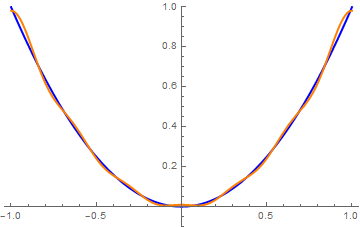As we see from the graph above, a six term approximation gives a relatively good representation of the given function. ■

Computing an integral in Mathematica is fairly painless, and it's tempting to simply use a partial Fourier sum depending to the number of terms n.

Example 6: Let's consider the following half-wave rectifier of the function cosx on interval [−π/2, ;π/2]:

$f(x) = \begin{cases} \cos x, & \ \mbox{ when } \ -\frac{\pi}{2} \le x \le \frac{\pi}{2} , \\ 0 , & \ \mbox{ when } \ \frac{\pi}{2} \le x \le \frac{3\pi}{2} . \end{cases}$
Outside the interval of length 2π, the function f(x) is expanded periodically.
f[t_] = Piecewise[{{Cos[t], -Pi/2 < t < Pi/2}, {0, -Pi < t < -Pi/2 && Pi/2 < t < Pi}}]
Out= { Cos[t] - Pi/2 < t < Pi/2
{ 0 True
Plot[f[t], {t, -Pi, Pi}, PlotStyle -> Thick]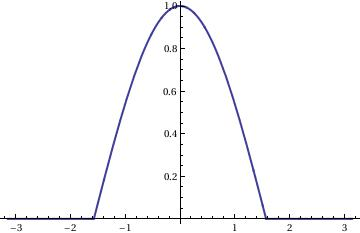Mathematica will treat the function f[t] outside the interval [-π , π ] as zero

Plot[f[t], {t, -3*Pi, 3*Pi}, PlotStyle -> Thick]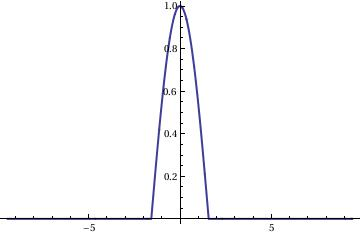As we see, Mathematica rescales vertical and horizontal lines so that the picture becomes almost symmetric. You can return to the proper picture by using AspectRatio option:

Plot[f[t], {t, -3*Pi, 3*Pi}, PlotStyle -> Thick, AspectRatio -> 1/3/Pi, Ticks -> {True, None}]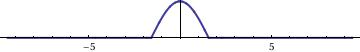So we get exactly the same graph. Now we are ready to expand this function into Fourier series by finding its Fourier coefficients:

\begin{align*} a_0 &= \frac{1}{\pi} \int_{-\pi /2}^{\pi /2} \cos x\,{\text d} x = \frac{2}{\pi} , \\ a_k &= \frac{1}{\pi} \int_{-\pi /2}^{\pi /2} \cos x\, \cos \left( k\, x \right) {\text d} x = -\frac{2}{\pi}\, \frac{1}{k^2 -1} \, \cos \frac{k\pi}{2} , \qquad k= 2, 3, \ldots ; \\ b_k &= \frac{1}{\pi} \int_{-\pi /2}^{\pi /2} \cos x\, \sin \left( k\, x \right) {\text d} x =0, \qquad k= 1, 2, 3, \ldots . \end{align*}
Then the corresponding Fourier series becomes
$f(t) \,=\,\frac{1}{\pi} + \frac{1}{2}\, \cos t - \frac{2}{\pi} \, \sum_{k\ge 1} \frac{1}{k^2 -1} \,\cos \left( k\, x \right) .$
A partial sum with N = 20 terms gives a very good approximation:We show how these commands work with other examples.

Example 7: We consider a piecewise continuous function that cannot be extended into Taylor series because it is identically zero on the interval (1,2). However, we can find its Fourier series.

f[x_] = Piecewise[{{1 - x, 0 < x < 1}, {0, 1 < x < 2}}]
Out= { 1-x, if 0<x<1 and 0 for 1<x<2.

The standard Mathematica command FourierTrigSeries provides you the Fourier series of the function that is extended periodically from the standard interval (-π,π):

FourierTrigSeries[f[x], x, 3]
Out= 1/(4 $Pi]) + (2 Cos[x] Sin[1/2]^2)/\[Pi] + (Cos[2 x] Sin^2)/(2 \[Pi]) + (2 Cos[3 x] Sin[3/2]^2)/( 9 \[Pi]) + ((2 - 2 Sin) Sin[x])/(2 \[Pi]) - ((-2 + Sin) Sin[2 x])/(4 \[Pi]) - ((-3 + Sin) Sin[3 x])/(9 \[Pi]) With option FourierParameters, it is equivalent to FourierTrigSeries[f[x], x, 3, FourierParameters -> {1, 1}] We can plot the partial sum with say 50 terms. Since Fourier partial sums oscillate near points of discontinuity exhibiting so called Gibbs phenomenon, we indicate maximum wiggle (overshoot) and minimum one: curve = FourierTrigSeries[f[x], x, 50]; Plot[curve, {x, -1.5, 13.5}, PlotRange -> {-0.3, 1.3}, Ticks -> {{Pi, 2*Pi, 3*Pi}, {1.09, -0.09}}, PlotStyle -> Thick]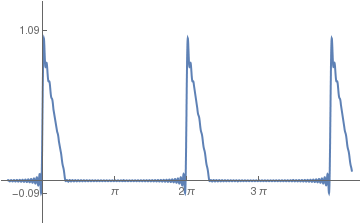However, it is not what we want: we need the Fourier series of the function f[x] extended periodically from the interval of length 2: FourierTrigSeries[f[x], x, 3, FourierParameters -> {1, Pi}] Out= 1/4 + (2 Cos[\[Pi] x])/\[Pi]^2 + (2 Cos[3 \[Pi] x])/(9 \[Pi]^2) + Sin[\[Pi] x]/\[Pi] + Sin[2 \[Pi] x]/(2 \[Pi]) + Sin[3 \[Pi] x]/( 3 \[Pi]) To check, we find the Fourier coefficients manually: Integrate[f[x]*Cos[n*Pi*x], {x, 0, 2}, Assumptions -> Element[n, Integers]] Out= (1 - Cos[n \[Pi]])/(n^2 \[Pi]^2) So \[ a_0 = \frac{1}{2} , \qquad a_n = \frac{1- (-1)^n}{n^2 \pi^2} , \quad n=1,2,\ldots .$
Integrate[f[x]*Sin[n*Pi*x], {x, 0, 2}, Assumptions -> Element[n, Integers]]
Out= (n $Pi] - Sin[n \[Pi]])/(n^2 \[Pi]^2) which is 1/(n*π). Hence, its Fourier series becomes \[ f(x) = \frac{1}{4} + \sum_{n\ge 1} \left[ \frac{1- (-1)^n}{n^2 \pi^2} \, \cos \left( n \pi x \right) + \frac{1}{n\pi}\, \sin \left( n \pi x \right) \right]$
We can also represent f(x) in other forms:
$f(x) = \frac{1}{4} + \sum_{n\ge 1} \sqrt{ \left( \frac{1- (-1)^n}{n^2 \pi^2} \right)^2 + \frac{1}{n^2 \pi^2}} \, \sin \left( n \pi x + \arctan \frac{1- (-1)^n}{n \pi} \right) = \frac{1}{4} + \sum_{n\ge 1} \sqrt{ \left( \frac{1- (-1)^n}{n^2 \pi^2} \right)^2 + \frac{1}{n^2 \pi^2}} \, \cos \left( n \pi x - \mbox{arccot} \frac{1- (-1)^n}{n \pi} \right) .$
Now we plot some partial sums, starting with the given function itself:

Plot[f[x], {x, -1, 3}, PlotRange -> {0, 1}, AspectRatio -> Automatic, Axes -> {True, False}, PlotStyle -> Thick]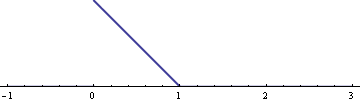Then we plot some finite sums:

curve5Pi = FourierTrigSeries[f[x], x, 5];
Plot[%, {x, -1, 3}]Then we repeat calculations with 50 terms:Next we plot the correct Fourier series partial sums:

curve5=FourierTrigSeries[f[x], x, 50, FourierParameters -> {1, Pi}];
Plot[%, {x, -1, 3}]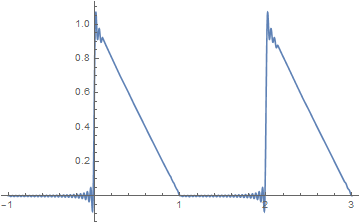The option FourierParameters has two parameters and when applied, it looks as FourierParameters->{a,b}
In trigonometric form, with setting $${\bf FourierParameters} \,-> \,\{ a, b \}$$ the following series is returned:

$\left\vert \frac{b}{\pi} \right\vert^{(1+a)/2} \left[ \frac{a_0}{2} + \sum_{k=1}^n \left( a_k \,\cos (bkt) + b_k \,\sin (bkt) \right) \right] ,$
with
$a_k = \left\vert \frac{b}{\pi} \right\vert^{(1+a)/2} \,\int_{-\pi/|b|}^{\pi/|b|} f(t)\,\cos (bkt) \,{\text d}t , \qquad k=0,1,2,\ldots ,$
$b_k = \left\vert \frac{b}{\pi} \right\vert^{(1+a)/2} \,\int_{-\pi/|b|}^{\pi/|b|} f(t)\,\sin (bkt) \,{\text d}t , \qquad k=1,2,\ldots .$

Syntax for the FourierSeries command is: FourierSeries [ function , variable , number of terms ]

Changing the FourierParameters setting allows control over the limits of integration on the coefficients, and therefore, the base frequency of the series. Some work will go into calculating what value for “b” will give the proper limits of integration. There is ordinarily no reason to change “a” from its default setting, which is 1.

Here are two important applications of Fourier series:
$\cos \left( a\,\sin x \right) = J_0 (a) + \sum_{k\ge 1} J_{2k} (a) \,\cos \left( 2kx \right) .$
$\delta (x-a) = \frac{1}{2\pi} \, \int_{-\infty}^{\infty} e^{{\bf j} (x-a)t} \, {\text d} t = \lim_{n\to \infty} \, \frac{1}{2\pi} \, \sum_{k=-n}^n \, e^{{\bf j} k(x-a)} \quad \left( = \frac{\sin \left[ \left( n+ \frac{1}{2} \right) (x-a) \right]}{2\pi\, \sin \frac{x-a}{2}} \right) ,$
where j is a unit vector in positive vertical direction on the complex plane, so $${\bf j}^2 =-1 .$$

We show how these commands work with other examples.

Example 8: Consider the piecewise continuous function on the interval [-2,2]:

$f(x) = \begin{cases} 1, & \ \mbox {if } -2 < x < -1 , \\ x^2 -1, & \ \mbox {if } -1< x< 2 , \end{cases}$
Its Fourier coefficients are evaluated with the aid of Mathematica:
f[x_] = Piecewise[{{1, -2 < x < -1}, {x^2 - 1, -1 < x < 2}}, 0]
Integrate[f[x], {x, -2, 2}]/2
1/2
Other coefficients we find by direct integration:
Simplify[Integrate[f[x]*Sin[n*Pi*x/2], {x, -2, 2}]/2 , Assumptions -> Element[n, Integers]]
-((2 (-1)^n (-4 + n^2 Pi]^2) + (8 + n^2 \[Pi]^2) Cos[(n \[Pi])/2] + 4 n \[Pi] Sin[(n \[Pi])/2])/(n^3 \[Pi]^3)) Simplify[Integrate[f[x]*Cos[n*Pi*x/2], {x, -2, 2}]/2 , Assumptions -> Element[n, Integers]] (8 (-1)^n n \[Pi] + 4 n \[Pi] Cos[(n \[Pi])/2] - (8 + n^2 \[Pi]^2) Sin[(n \[Pi])/ 2])/(n^3 \[Pi]^3) So \begin{align*} a_0 &= \frac{1}{2} , \\ a_n &= \frac{8(-1)^n n\pi + 4n\pi \cos \left( \frac{n\pi}{2} \right) - \left( 8 + n^2 \pi^2 \right) \sin \left( \frac{n\pi}{2} \right)}{n^3 \pi^3} , \\ b_n &= - \frac{2 (-1)^n \left( n^2 \pi^2 -4 \right) + \left( 8 + n^2 \pi^2 \right) \cos \left( \frac{n\pi}{2} \right) + 4n\pi\,\sin \left( \frac{n\pi}{2} \right)}{n^3 \pi^3} . \end{align*} Finally, we build partial Fourier sums with N = 10, 20, and 100 terms F10[x_] = 1/4 + Sum[(( 8 (-1)^n n \[Pi] + 4 n \[Pi] Cos[(n \[Pi])/2] - (8 + n^2 \[Pi]^2) Sin[(n \[Pi])/ 2])/(n^3 \[Pi]^3))* Cos[n*Pi*x/2] - (( 2 (-1)^n (-4 + n^2 \[Pi]^2) + (8 + n^2 \[Pi]^2) Cos[(n \[Pi])/ 2] + 4 n \[Pi] Sin[(n \[Pi])/2])/(n^3 \[Pi]^3))* Sin[n*Pi*x/2], {n, 1, 10}] F20[x_] = 1/4 + Sum[(( 8 (-1)^n n \[Pi] + 4 n \[Pi] Cos[(n \[Pi])/2] - (8 + n^2 \[Pi]^2) Sin[(n \[Pi])/ 2])/(n^3 \[Pi]^3))* Cos[n*Pi*x/2] - (( 2 (-1)^n (-4 + n^2 \[Pi]^2) + (8 + n^2 \[Pi]^2) Cos[(n \[Pi])/ 2] + 4 n \[Pi] Sin[(n \[Pi])/2])/(n^3 \[Pi]^3))* Sin[n*Pi*x/2], {n, 1, 20}] F100[x_] = 1/4 + Sum[(( 8 (-1)^n n \[Pi] + 4 n \[Pi] Cos[(n \[Pi])/2] - (8 + n^2 \[Pi]^2) Sin[(n \[Pi])/ 2])/(n^3 \[Pi]^3))* Cos[n*Pi*x/2] - (( 2 (-1)^n (-4 + n^2 \[Pi]^2) + (8 + n^2 \[Pi]^2) Cos[(n \[Pi])/ 2] + 4 n \[Pi] Sin[(n \[Pi])/2])/(n^3 \[Pi]^3))* Sin[n*Pi*x/2], {n, 1, 100}] and plot them: Plot[{f[x], F10[x]}, {x, -2.5, 2.5}, PlotStyle -> {{Thick, Black}, {Thick, Red}}] Plot[{f[x], F20[x]}, {x, -2.5, 2.5}, PlotStyle -> {{Thick, Black}, {Thick, Orange}}] Plot[{f[x], F100[x]}, {x, -2.5, 2.5}, PlotStyle -> {{Thick, Black}, {Thick, Blue}}] Fourier approximation with 10 terms Fourier approximation with 20 terms Fourier approximation with 100 terms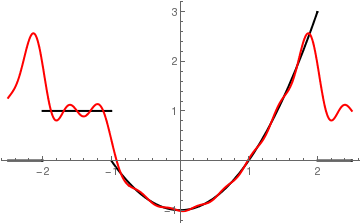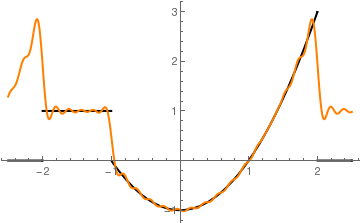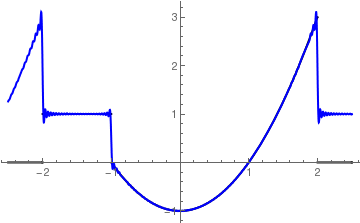■ Example 9: Let us consider a periodic step function with period T: \[ f(x) = \mbox{sign}\left( \sin \frac{2\pi x}{T} \right) = \begin{cases} \phantom{-}1, & \ \mbox{for }\ 0 < x < T /2, \\ -1, & \ \mbox{for }\ T/2 < x < T . \end{cases}
Plot[Sign[Sin[Pi*x]], {x, 0, 4}, PlotStyle -> Thick]
Its Fourier series becomes
$\mbox{sign}\left( \sin \frac{2\pi x}{T} \right) = \frac{4}{\pi} \sum_{n\ge 1} \frac{1}{2n-1}\,\sin \frac{2\pi x \left( 2n -1 \right)}{T} .$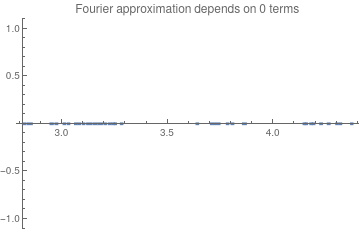Using Mathematica We plot animation of the Fourier series approximation depending on the number m of terms. s[x_, m_] = 4*Sum[Sin[Pi*x*(2*n - 1)]/(2*n - 1), {n, 1, m}]/Pi; animation = Table[Plot[s[x, m], {x, -0.1, 2*Pi + 0.1}, PlotRange -> {-1, 1, 1.1}, PlotStyle -> Thickness[0.008], PlotLabel -> "Fourier approximation depends on " <> ToString[m] <> " terms"], {m, 0, 100, 2}];; Export["Animation.gif", animation, "AnimationRepetitions" -> 100] Fourier series approximation depending on the number of terms. . Mathematica code

■

Term-by-term differentiation of infinite series

Not every Fourier series can be term-by-term differentiated, but those that correspond to functions having derivatives expanded into Fourier series. Term-by-term differentiation is not justified for functions having a jump discontinuity.

Theorem 7: If the derivative f'(x) of a function f(x) is piecewise smooth on [−ℓ, ;ℓ] and f(−ℓ) = f(ℓ), then a continuous Fourier series of f(x) can be differentiated term by term.

Example 10: Let us consider a function f(x) = x on the interval [0, ℓ]. Its Fourier series is

$x = \sum_{n\ge 1} \frac{2\ell}{n\pi} \,(-1)^{n+1} \,\sin \left( \frac{n\pi x}{\ell} \right) , \qquad \ell < x < \ell .$
You will learn later in the even and odd section how to construct this series and determine the coefficients, but now you have to trust me or plot partial sums to verify the identity. If we differentiate the function on the left-hand side, then we get the function 1. However, if we formally differentiate term by term the function on the right, then we arrive at
$1 \stackrel{?}{=} 2 \sum_{n\ge 1} (-1)^{n+1} \,\cos \left( \frac{n\pi x}{\ell} \right) , \qquad \ell < x < \ell .$
The series at the left does not converge because its general term does not tern to zero. However, we will see later that if we use another definition of convergence, called Cesàro summation, the right-hand side series converges to 1. If we formally apply geometric series formula (see Example 2 in Cesàro summation section)
$\sum_{n\ge 1} q^n = \frac{q}{1-q} , \qquad \mbox{with} \quad q = -e^{{\bf j}x} ,$
we obtain
\begin{align*} \sum_{n\ge 1} (-1)^{n+1} \,\cos \left( nx \right) &= - \Re \sum_{n\ge 1} (-1)^{n} \, e^{{\bf j}nx} = - \Re \sum_{n\ge 1} \left( -e^{{\bf j}x} \right)^n - \\ &= - \Re \frac{-e^{{\bf j}x}}{1 + e^{{\bf j}x}} = - \Re \frac{-e^{{\bf j}x} \left( 1 + e^{-{\bf j}x} \right)}{\left( 1 + e^{{\bf j}x} \right) \left( 1 + e^{-{\bf j}x} \right)} = - \Re \frac{- e^{{\bf j}x} -1}{2 + 2\,\cos x} \\ &= \Re \frac{1 + e^{{\bf j}x}}{2 \left( 1 + \cos x \right)} = \frac{1 + \cos x}{2 + 2\,\cos x} = \frac{1}{2} . \end{align*}

However, wait a minute. There exist another Fourier series for the same function (if you open the same section):
$x = \frac{\ell}{2} - \frac{4\ell}{\pi^2} \sum_{n\ge 1} \frac{1}{n^2} \,\cos \left( \frac{n\pi x}{\ell} \right) , \qquad \ell < x < \ell .$
Fourier sine series of the derivative f'(x) = 1 can be obtained by term-by-term differentiation of the Fourier cosine series of f(x) = x. Assuming that term-by-term differentiation is valid as claimed, it follows that
$1 = \frac{4}{\pi} \sum_{n\ge 1} \frac{1}{n}\, \sin \left( \frac{n\pi x}{\ell} \right) , \qquad \ell < x < \ell ,$
which is, in fact, correct.    ■

Product of Fourier series

Suppose we have two Fourier sum-functions f(x) and g(x). When these functions are defined by trigonometric series \eqref{EqFourier.5}, there is no suitable formula to determine the Fourier coefficients for their product f g(x). However, when these functions are expanded into complex Fourier series
$f(x) \,\sim\, \sum_{k=-\infty}^{\infty} \alpha_k e^{k{\bf j} \pi x/\ell} , \qquad g(x) \,\sim\, \sum_{k=-\infty}^{\infty} \beta_k e^{k{\bf j} \pi x/\ell} ,$
then their product is
$f(x)\,g(x) \,\sim\, \sum_{k=-\infty}^{\infty} \gamma_k e^{k{\bf j} \pi x/\ell} ,$
where
$\gamma_n = \sum_{j=-\infty}^{\infty} \alpha_j \beta_{n-j}$
is known as the convolution of two Fourier series.

1. Carleson, L., On convergence and growth of partial sums of Fourier series, Acta. Math. 116 (1966), pp. 135–137.
2. Gibbs, J. W., Fourier Series, Nature, 1899, Vol. 59, 200 and 606.
3. Hunt, R., On the convergence of Fourier series, Orthogonal Expansions and their Continuous Analogues (Proc. Conf. Edwardsville, IL, 1967), Southern University Press, Carbondale, IL, 1968, pp. 235–255.
4. R. Hunt and M. Taibleson, Almost everywhere convergence of Fourier series on the ring of integers of a local field, SIAM J. Math. Anal. 2 (1971), pp. 607–625.
5. Körner, T.W., Fourier Analysis, Cambridge University Press; 1 edition (January 28, 1988).
6. Tolstov, G.P., Fourier Series, Dover Publications, 2012.
7. Walker, J.S., Fourier Analysis, 1988, Oxford University Press, New York.